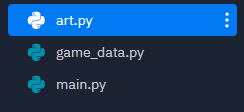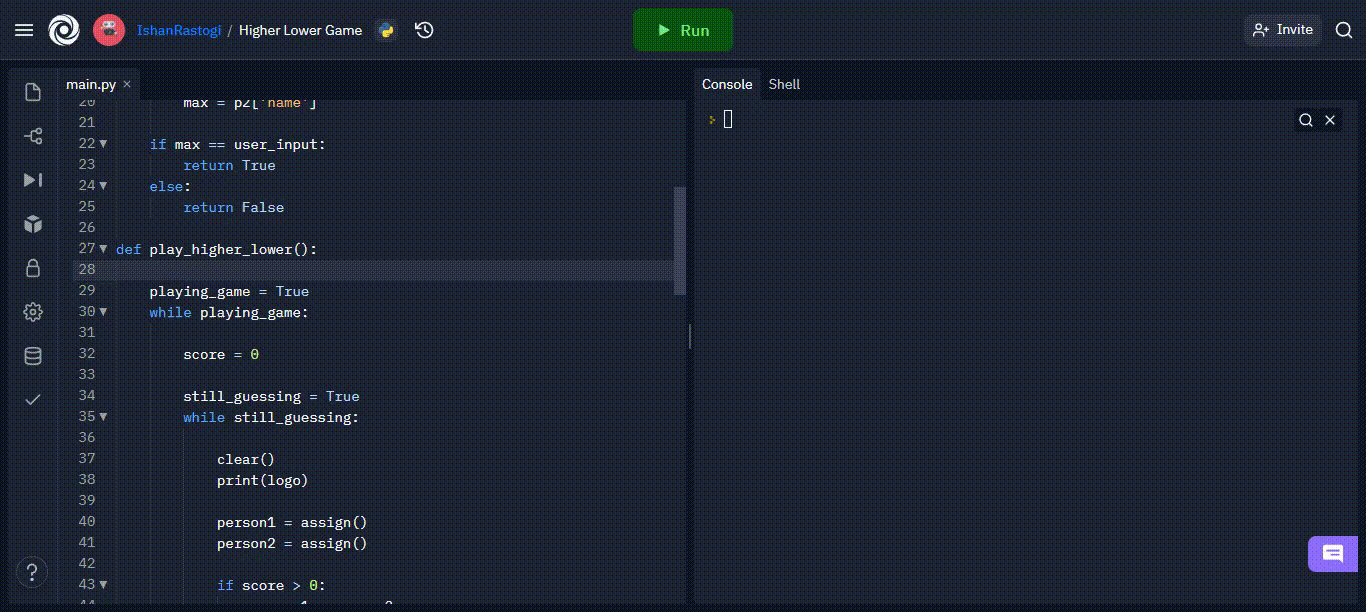# Higher-Lower Game with Python

In this article, we will be looking at the way to design a game in which the user has to guess which has a higher number of followers and it displays the scores.

## Game Play:

The name of some Instagram accounts will be displayed, you have to guess which has a higher number of followers by typing in the name of that account. Make sure you type the name exactly as shown. If the answer is correct, one of the accounts will stay, and some other account will be displayed to compare to. We will develop the game using basic concepts of Python.

Project StructureAdditional Resources – We will use some ASCII art to show the title of our game and make it look more attractive. It will be stored in a separate python file – art.py. You can generate your own ASCII art from here: https://patorjk.com/software/taag/#p=display&f=Graffiti&t=Type%20Something%20

File: art.py

## Python3

 `# three quotation marks are used to make a multiline string.`   `logo ``=` `"""` `  ``_    _ _       _                 _                            ` ` ``| |  | (_)     | |               | |                           ` ` ``| |__| |_  __ _| |__   ___ _ __  | |     _____      _____ _ __ ` ` ``|  __  | |/ _` | '_ \ / _ \ '__| | |    / _ \ \ /\ / / _ \ '__|` ` ``| |  | | | (_| | | | |  __/ |    | |___| (_) \ V  V /  __/ |   ` ` ``|_|  |_|_|\__, |_| |_|\___|_|    |______\___/ \_/\_/ \___|_|   ` `            ``__/ |                                               ` `           ``|___/                                                ` `"""`     `vs ``=` `"""` ` ``_    __    ` `| |  / /____` `| | / / ___/` `| |/ (__  ) ` `|___/____(_)` `"""`

## Game Data :

The next part is to have some data for our game to work. Note that the data is bound to be incorrect as compared to when you are reading this article as the number of followers keeps changing. So to have highly accurate data, you update it yourself. Game data is simply a Python list of dictionaries. You can find sample data in this file game_data Copy it and paste it into a separate python file – game_data.py. This is what a small sample of complete data will look like.

File: game_data.py

## Python3

 `data ``=` `[` `    ``{` `        ``'name'``: ``'Instagram'``,` `        ``'follower_count'``: ``346``,` `        ``'description'``: ``'Social media platform'``,` `        ``'country'``: ``'United States'` `    ``},` `    ``{` `        ``'name'``: ``'Cristiano Ronaldo'``,` `        ``'follower_count'``: ``215``,` `        ``'description'``: ``'Footballer'``,` `        ``'country'``: ``'Portugal'` `    ``},` `    ``{` `        ``'name'``: ``'Ariana Grande'``,` `        ``'follower_count'``: ``183``,` `        ``'description'``: ``'Musician and actress'``,` `        ``'country'``: ``'United States'` `    ``}` `]`

## Game Logic:

### Step 1: First, we need to make some necessary imports:

Data stored in a Python file can be used in some other Python file using from and import keyword where from specifies from which file we want to import and import specifies which data we want to use. We also make an optional import, which is a clear() method, that will clear out the output console, so that the game can be carried out for a longer period without cluttering the output screen with unnecessary output text.

## Python3

 `# since we want to randomly select an ` `# option from the data, we need random ` `# module` `import` `random`   `# import the game data` `from` `game_data ``import` `data`   `# import the ASCII art to display.` `from` `art ``import` `logo` `from` `art ``import` `vs`   `# IDEs like Replit have their own "clear" ` `# functions to clear the output console:` `from` `replit ``import` `clear`   `# If you are using some other IDE, this import ` `# will not work.` `# You will have to use clear of that IDE` `# For example, in Google Colab Notebook we have:` `from` `googl.colab ``import` `output`

### Step 2: Create three functions, to play the game, select an option from game_data, and compare the winner.

Data stored in a Python file can be used in some other Python file using from and import keyword where from specifies from which file we want to import and import specifies which data we want to use. We also make an optional import, which is a clear() method, that will clear out the output console, so that the game can be carried out for a longer period without cluttering the output screen with unnecessary output text.

## Python3

 `def` `assign():` `    ``pass`   `def` `compare(p1, p2, user_input):` `    ``pass`   `def` `play_higher_lower():` `    ``pass`     `want_to_play ``=` `input``(``"Do you want to play Higher Lower? (y/n)\n"``).lower()` `if` `want_to_play ``=``=` `'y'``:` `    ``clear()` `    ``play_higher_lower()` `elif` `want_to_play ``=``=` `'n'``:` `    ``print``(``"Program Exit Successful."``)` `else``:` `    ``print``(``"Invalid Input, Program Exited."``)`

### Step 3: Make the assign function work:

Here, the choice method of random modules randomly picks an item from a list. The item, which in this case is a dictionary, is stored in a variable wherever this function is called.

## Python3

 `def` `assign():` `    ``return` `random.choice(data)`

### Step 4: Make the compare function work:

We store the follower counts of each account in a variable and compare them to see which account has a greater number of follower counts and store the name of that account in a variable, max. Then we compare it with the user input name, if the user inputs the correct name, then True is returned otherwise False is returned.

## Python3

 `def` `compare(p1, p2, user_input):`   `    ``# store the follower count of` `    ``# account1 in a variable` `    ``sum1 ``=` `p1[``'follower_count'``]` `    `  `    ``# store the follower count of ` `    ``# account2 in a variable` `    ``sum2 ``=` `p2[``'follower_count'``]` `    `  `    ``# make an empty variable max, where` `    ``# we will store the name of account` `    ``# with highest followers, then compare` `    ``# it with user input name.` `    ``max` `=` `""` `    `  `    ``# if account1 has greater follower count` `    ``if` `sum1 > sum2:` `          `  `        ``# max is name of account1 ` `        ``max` `=` `p1[``'name'``]` `    ``elif` `sum1 < sum2:` `          `  `        ``# otherwise, if account2 has higher` `        ``# follower count, ` `        ``# max is name of account two.` `        ``max` `=` `p2[``'name'``]` `    `  `    ``# now compare the name of account with greater` `    ``#follower count against the user input name,` `    ``# if user is correct, return True` `    ``if` `max` `=``=` `user_input:` `        ``return` `True` `    ``else``:` `          ``# otherwise return False` `        ``return` `False`

### Step 5: Make the gameplay function work:

We need a loop to continuously keep asking for user input, and keep checking them. If he is correct, the loop will continue, otherwise loop break. We don’t want the function to end there, we want to ask him if would like to play again, we do this by using an outer while loop, which will keep running as long as the user wants to keep playing. In the loop for current gameplay, make two variables and use the assign method to assign them an account, display them, then ask for the user input, compare it and continue the loop if the user is correct. In the second iteration, we want the change the accounts displayed on the screen. We want to keep the second account, make it as account one, and use assign method to assign some other account to account2. To make this happen in the second iteration, and not in the first, we need to track the score, which initially is zero and increases if the user is correct. If the score is not zero then it means the user is not in the first iteration, and his previous answer was correct. Thus we change the accounts to display in this if check.

After the compare function is called, the score increase by 1 and the loop continues otherwise score is set to zero, and the game loop end. Due to the outer loop, the user is asked for input, if he wants to continue or not if he wants to, the loop continues, due to which a new game starts which will keep executing as long the user keeps entering correct input.

## Python3

 `def` `play_higher_lower():` `    `  `    ``# infinite loop to make user play ` `    ``# game again and again.` `    ``playing_game ``=` `True` `    ``while` `playing_game:` `        `  `        ``# variable to keep track fo suer's score, ` `        ``# i.e., how many times he answers correctly` `        ``score ``=` `0` `        `  `        ``# infinite loop to continue the current game play` `        ``still_guessing ``=` `True` `        ``while` `still_guessing:` `            `  `            ``# print the logo after clearing the screen.` `            ``clear()` `            ``print``(logo)` `            `  `            ``# assign two account names to display` `            ``person1 ``=` `assign()` `            ``person2 ``=` `assign()` `            `  `            ``# if we are not in first iteration, and user` `            ``# input corret answer to previous iteration,` `            ``# in that case, make account1 become account2` `            ``# and randomly asing account2 some other account.` `            ``if` `score > ``0``:` `                ``person1 ``=` `person2` `                ``person2 ``=` `assign()` `                `  `                ``# we need to make sure that the two accounts` `                ``# are not same.` `                ``if` `person1 ``=``=` `person2:` `                    ``person2 ``=` `assign()` `            `  `            ``# display account1 name and description` `            ``print``(f``"Name: {person1['name']}, Desc: {person1['description']}"``)` `            `  `            ``# display the "VS" art` `            ``print``(vs)` `            `  `            ``# display account2 name and description` `            ``print``(f``"Name: {person2['name']}, Desc: {person2['description']}"``)` `            `  `            ``# display current score` `            ``print``(``"----------------------------------------------"``)` `            ``print``(f``"Your current score is: {score}"``)` `            ``print``(``"----------------------------------------------"``)` `            `  `            ``# ask for user input` `            ``guess ``=` `input``(``"Enter name of person with Higher Followers: "``)` `            `  `            ``# see if user is correct` `            ``if` `compare(person1, person2, guess):` `              `  `                  ``# if user is correct continue to next iteration` `                ``# and increase score by 1` `                ``score ``+``=` `1` `            ``else``:` `                ``# if user is wrong, the current game play loop stops` `                ``still_guessing ``=` `False` `                `  `        ``# since the user was wrong in previous iteration and ` `        ``# the game ended, ask him if he want to play again.` `                `  `        ``play_again ``=` `input``(``"Want to Play Again? (y/n): "``).lower()` `        `  `        ``# if he want to, continue, otherwise end the outer ` `        ``# loop as well. also check if the user entered some` `        ``# other input than what is allowed` `        ``if` `play_again ``=``=` `'y'``:` `            ``continue` `        ``elif` `play_again ``=``=` `'n'``:` `            ``playing_game ``=` `False` `            ``clear()` `            ``print``(``"Game Exited Successfully"``)` `        ``else``:` `            ``playing_game ``=` `False` `            ``print``(``"Invalid Input Taken as no."``)`

Output:Whether you're preparing for your first job interview or aiming to upskill in this ever-evolving tech landscape, GeeksforGeeks Courses are your key to success. We provide top-quality content at affordable prices, all geared towards accelerating your growth in a time-bound manner. Join the millions we've already empowered, and we're here to do the same for you. Don't miss out - check it out now!

Previous
Next